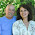Math trick and its proof: square a number ending in 5

I will be hosting the blog carnival Math Teachers at Play next week. (You can send in submissions here.)

One submission I got about various multiplication tricks or shortcuts got me inspired to write a proof of the particular trick.

You could definitely use this in algebra class. First explain the shortcut or trick itself. Then ask students to prove it, or to explain WHY it works, using algebra.

You could also explain this to younger students as an additional "neat trick" and let them explore and play with it.

THE "TRICK"

If a number ends in 5, then its square can be calculated using this "trick" (I like to call it a shortcut because there's nothing magic about it):

Let's say we have 75 × 75 => Go 7 × 8 = 56. Then tag 25 (or 5 × 5) into that. You get 5625.

Let's say we have 35 × 35 => Go 3 × 4 = 12. Then tag 25 into that. You get 1225.

Let's say we have 115 × 115 => Go 11 × 12 = 132. Then tag 25 into that. You get 13,225.

Let's say we have 245 × 245 => Go 24 × 25 = 600. Then tag 25 into that. You get 60,025.

So, you simply take the digit or digits in front of the 5 and consider those as a number in itself. Multiply that by the next number. Then "tag" 25 to the answer you got in the previous step.

PROOF

Any whole number that ends in five is of the form A + 5, and A is a multiple of 10. Since A is a multiple of 10, we can write A = 10b, where b is now some whole number. So, our number is of the form 10b + 5. Now, let's square it.

(10b + 5)(10b + 5) and we use the distributive property to multiply this out.

(10b + 5)(10b + 5) = 100b2 + 50b + 50b + 25 = 100b2 + 100b + 25

Now, notice those 100b's there. We can gather that as a common factor for the first two terms:

= 100 b (b + 1) + 25

This is now essentially in the form that the trick is using.

The trick says to take b, or the number formed by the digits in front of the 5. That corresponds exactly with our b! (For example, in number 645, b is 64. Our number 645 is 10b + 5, or 640 + 5.)

So we take b, multiply it by (b + 1) which is the next number, and also by 100, and lastly add 25.

Now, b × (b+1) is the part of the trick where you multiply the digits in front of the 5 by the next number. To "tag" 25 to those digits means you add 25 only after having multiplied the number by 100 so that it would end in "00". Once it ends in "00" you can add 25 (or any two-digit number) and it is the same as "tagging" 25 to the digits without the "00".

I hope this is clear enough.

By the way, I do not feel all students must learn this shortcut for finding the square of numbers ending in 5. It is a nice addition to one's mathematical knowledge, but not any necessity. However, it is useful as an algebra problem, and of course has been used as such over the course of centuries, I'm sure.Pat B said…
Marla,
This can be generalized to any numbers as long as the tens digits are the same and the last two are tens compliments... ie 75x75=5635 74x76 =564 and 73x77=5621...

and if you learn a simple rule for multiplying any two numbers in the teens, you can extend it up to 195x195
for example, to multiply 14x15 you add 14+5 (or 15+4) for the number of tens (ie 190) then and the product of hte last two digits (5x4=20) to get 210
s0 145x145=210___25

a second example, to do 175^3 we do 17x18 = 17+8 = 25 tens or 250 and then add 7x8=56 to get 306, now tack on the 25 to get 175^2=30625...
there is also an easy way to multiply numbers in the twentys..etc...Anonymous said…
the explanation of the 'trick' itself could use a little work - use a graphic program to step it out more clearly by circling the bits you are referring to at each step of the algorithm.

the proof is clear.Anonymous said…
I learned this trick in High School. Thanks for the formal proof.Bill James said…
Glad you liked the post. That's the best thing about math tricks, is they're all provable, or they wouldn't work!